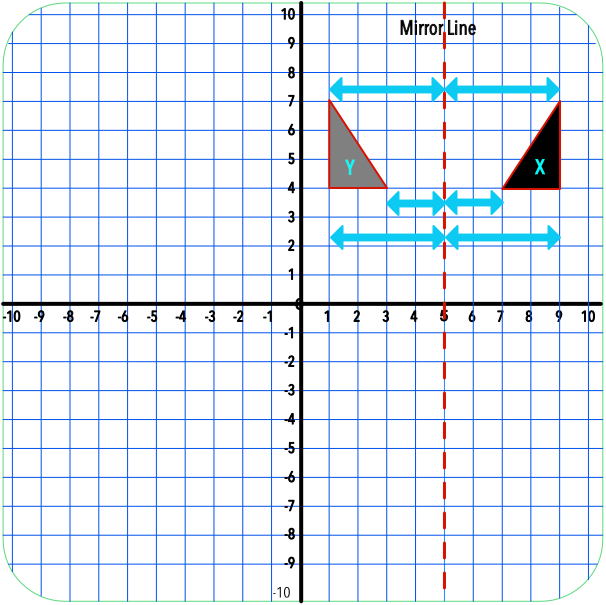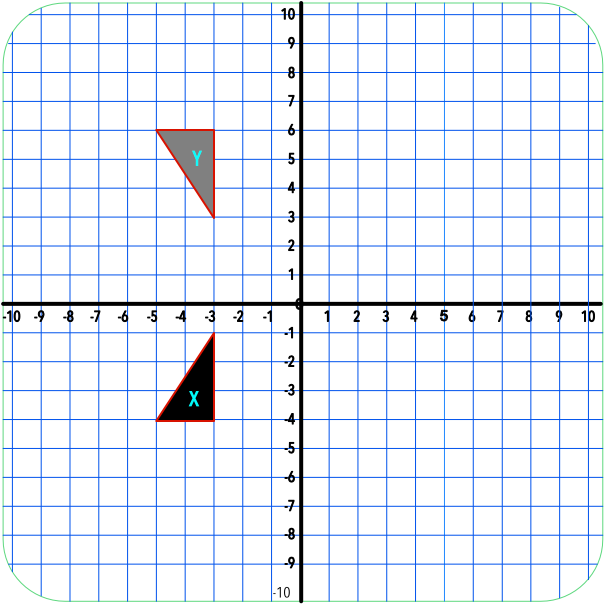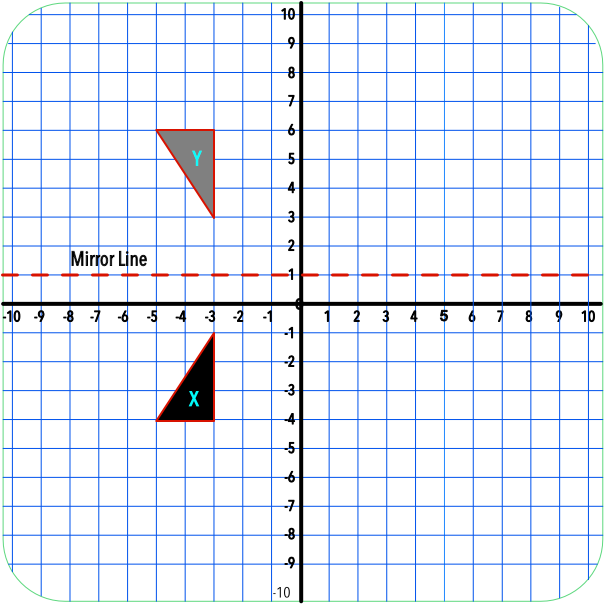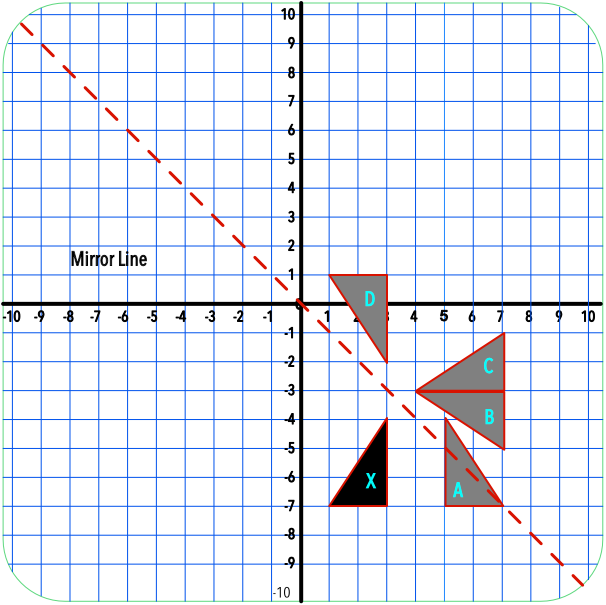Reflection

# Reflection

GCSE(F), GCSE(H),

A Reflection is a transformation. A reflection requires a Mirror Line. Reflections may be shown on a grid, and the mirror line given as an equation.

Given a mirror line, all the vertices, or corners, of the shape are always the same distance from the mirror line in the reflected image as they are in the original image.

To find a mirror line, locate the mid-point between each set of vertices, and draw the mirror line through these points.## Examples

1. Describe fully the transformation that maps shape X onto shape Y.Answer: A reflection in the line y = 1

The vertical distance between the nearest points of the two images is 4 grid spaces; the mirror line lies halfway along that line. Repeat for the other vertices to determine the reflection line y = 1.2. A shape X is reflected in the line x = -y. What is the position of the reflected shape: A, B, C or D?